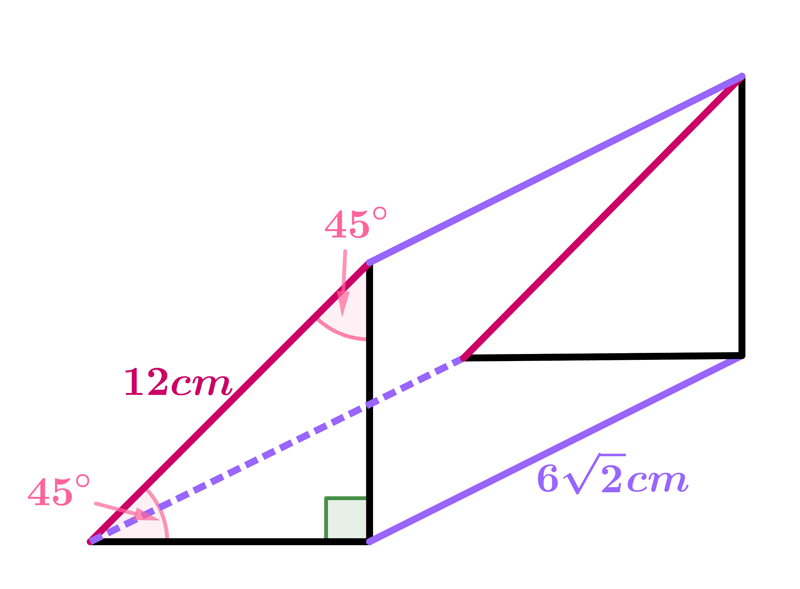Math Calculators, Lessons and Formulas

It is time to solve your math problem

mathportal.org
• Solid figures
• Cube, cuboid and Prism
• Triangular prism

# Triangular prism

ans:
syntax error
C
DEL
ANS
±
(
)
÷
×
7
8
9
4
5
6
+
1
2
3
=
0
.
auto next question
calculator
•  Question 1: 1 pts Find the surface area of the right, regular, triangular prism shown on the picture.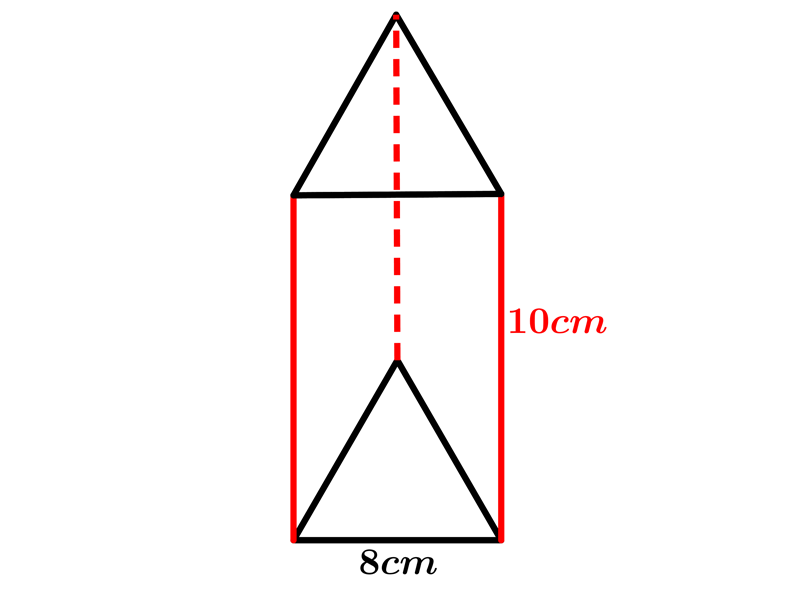$24\cdot (6+2\sqrt{3})cm^{2}$ $16\cdot (15+2\sqrt{3})cm^{2}$ $8\cdot (15+\sqrt{3})cm^{2}$ $18\cdot (10+\sqrt{3})cm^{2}$
•  Question 2: 1 pts Find the surface area of the right, regular, triangular prism if the perimeter of its base is $12cm.$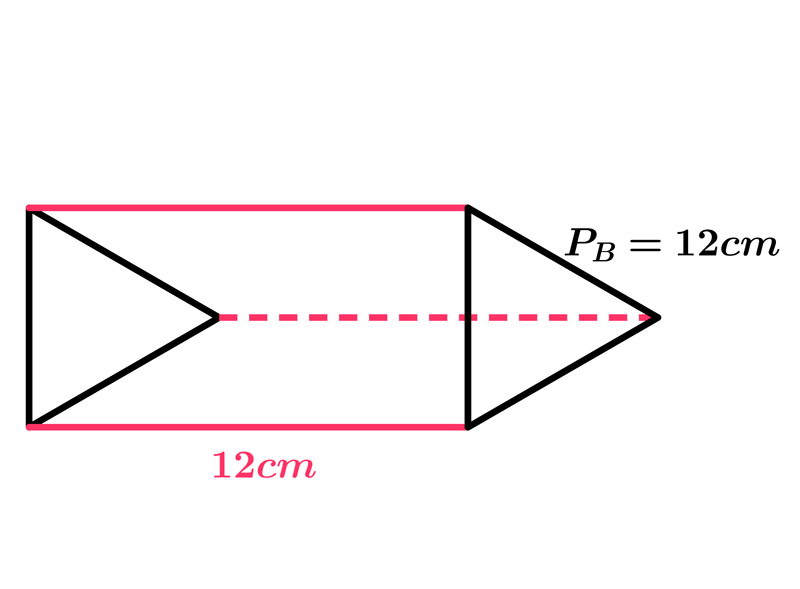$6\cdot (6+2\sqrt{3})cm^{2}$ $6\cdot (8+2\sqrt{3})cm^{2}$ $8\cdot (15+\sqrt{3})cm^{2}$ $8\cdot (18+\sqrt{3})cm^{2}$
•  Question 3: 1 pts Find the volume of this right, regular, triangular prism if the surface area of its base is $\dfrac{9\sqrt{3}}{4}cm^{2}.$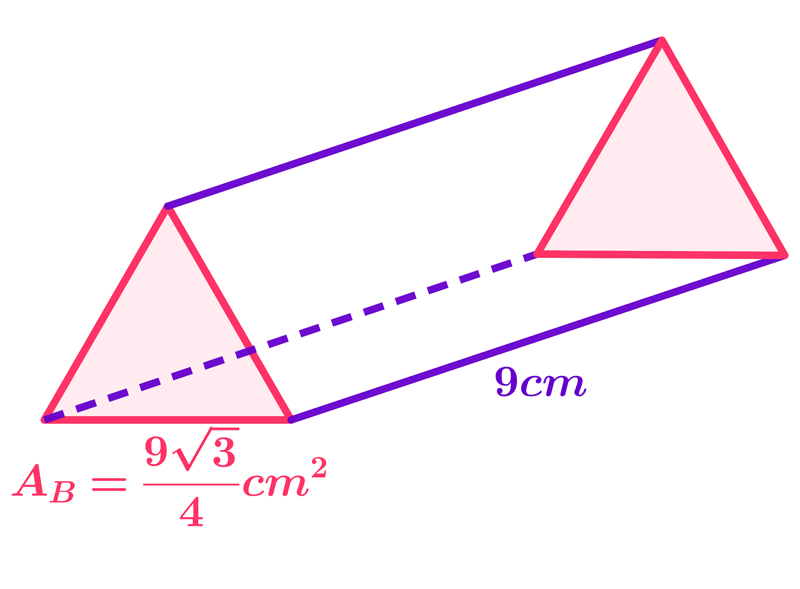$\dfrac{16\sqrt{2}}{3}cm^{2}.$ $\dfrac{63\sqrt{3}}{4}cm^{2}.$ $\dfrac{81\sqrt{3}}{4}cm^{2}.$ $\dfrac{9\sqrt{3}}{4}cm^{2}.$
•  Question 4: 1 pts Find the volume of the right, regular, triangular prism whose basic edge is $16 cm$ long, and the area of total lateral surface is $48 cm ^ {2}$
 $64\sqrt{3}cm^{3}$ $28\sqrt{3}cm^{3}$ $16\sqrt{3}cm^{3}$ $8\sqrt{3}cm^{3}$
•  Question 5: 2 pts Find the surface area of the right, regular triangular prism whose volume is $3\sqrt{3} cm^{3},$ and the height of the prism is $3cm$
 $4\cdot (\sqrt{3}+4)cm^{2}$ $2\cdot (\sqrt{3}+9)cm^{2}$ $3\cdot (\sqrt{2}+9)cm^{2}$ $4\cdot (\sqrt{2}+6)cm^{2}$
•  Question 6: 2 pts Find the surface area of the right, regular triangular prism whose base edge is equal to its height, and the sum of all edges is $54cm.$
 $6\cdot (\sqrt{2}+6)cm^{2}$ $8\cdot (\sqrt{3}+4)cm^{2}$ $18\cdot (\sqrt{3}+6)cm^{2}$ $2\cdot (\sqrt{2}+8)cm^{2}$
•  Question 7: 2 pts The base of a right, regular prism is a triangle whose area is 16\sqrt{3}cm^{2}, and the area of total lateral surface is $48 cm ^{2}.$ Find the volume of that prism.
$V=$
•  Question 8: 2 pts The surface area of right, regular triangular prism is $10\cdot (5\sqrt{3}+16)cm^{2}$, and the length of base edge is 10cm. Find the volume of that prism.
 $\dfrac{625\sqrt{3}}{3}cm^{3}$ $\dfrac{425\sqrt{3}}{2}cm^{3}$ $\dfrac{400\sqrt{3}}{3}cm^{3}$ $\dfrac{200\sqrt{3}}{3}cm^{2}$
•  Question 9: 3 pts The base of a right prism is a triangle whose sides arc of lengths $13 cm, 20 cm$ and $21 cm.$ If the height of the prism be $9 cm$ find the volume of the prism.
 $1134cm^{3}$ $996cm^{3}$ $625cm^{3}$ $343cm^{3}$
•  Question 10: 3 pts Find the surface area of the right, regular, triangular prism shown on the picture if the prism height is equal to the hypothesis of the triangle in the basis.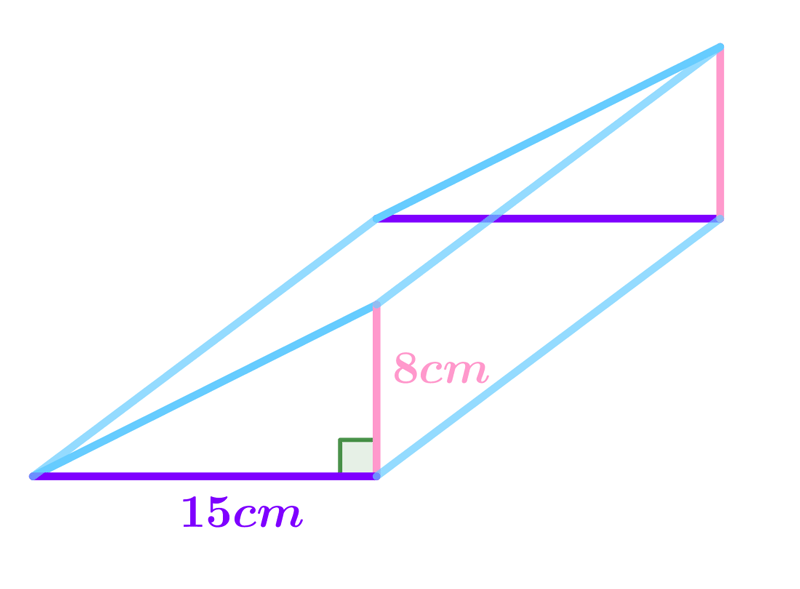$A=$
•  Question 11: 3 pts The following expression can be used to find the surface area of the triangular prism shown on the picture $$A=\left(\dfrac{10\cdot 8}{2}+3\cdot12 \cdot 10\right)cm^{2}$$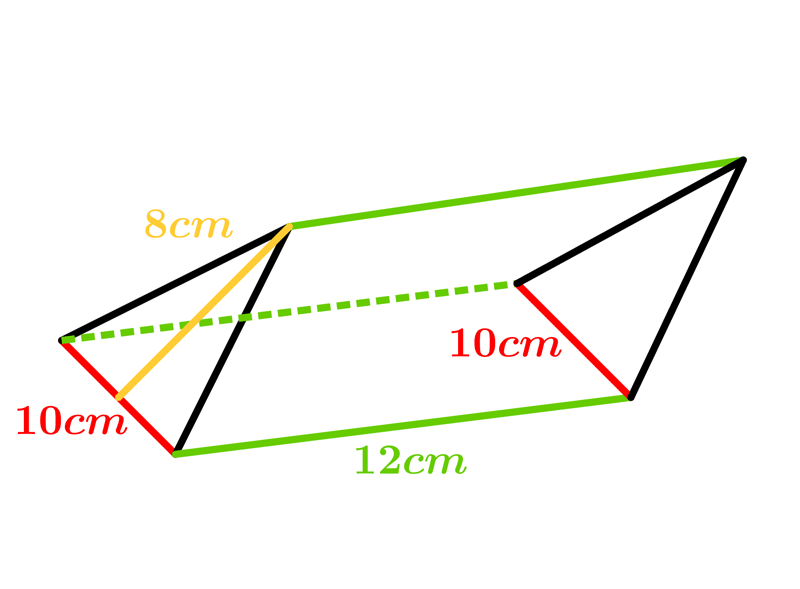•  Question 12: 3 pts The following expression can be used to find the surface area of the right, regular, triangular prism shown on the picture $$A=2\cdot \dfrac{6\sqrt{2}\cdot6\sqrt{2}}{2}+12\cdot 6\sqrt{2}+2\cdot 6\sqrt{2}\cdot 6\sqrt{2}=72\cdot \left(\sqrt{2}+3\right)cm^{2}.$$